# Extreme Indices

The extreme indices are an ensemble of indices based on the Expert Team on Climate Change Detection Indices (ETCCDI). There are currently 5 available indices to be computed: extreme heat (tx90p), extreme cold (tn10p), extreme wind (wx), drought (ccd) and flooding (rx5day). The individual indices can be combined into a single index with or without weighting for each component.

This example requires the following system libraries:

• libssl-dev
• libnecdf-dev
• cdo

The ClimProjDiags R package should be loaded by running the following lines in R once it’s integrated into CRAN mirror.

library(ClimProjDiags)

All the other R packages involved can be installed directly from CRAN and loaded as follows:

library(s2dverification)
library(abind)
library(multiApply)
library(ggplot2)
library(parallel)

### 2- Synthetic data

Daily maximum and minimum temperature, wind speed and precipitation are necessary to compute the different indices in both, the reference period (1971 - 2000) and the future projection (2006 - 2100). The defined region will be in the northern hemisphere between -40 - 20 ºE and 25 - 60 ºN.

Maximum temperature is generated considering the annual cycle:

lat <- seq(25, 60, 5)
lon <- seq(-35, 20 ,5)
tmax_historical <- NULL
grid1 <- 293 - 10 * cos(2 * pi / 365 * (1 : 10958)) + rnorm(10958)
gridlon <- NULL
for (i in 1 : 12) {
gridlon <- cbind(gridlon,
grid1 + rnorm(10958, sd = 5) * cos(2 * pi / 365 * (1 : 10958)))
}
for (j in 1 : 8) {
gridnew <- apply(gridlon, 2, function(x) {x - rnorm(10958, mean = j * 0.5, sd = 3)})
tmax_historical <- abind(tmax_historical, gridnew, along = 3)
}
tmax_historical <- InsertDim(InsertDim(tmax_historical, posdim = 1, lendim = 1),
posdim = 1, lendim = 1)
names(dim(tmax_historical)) <- c("model", "var", "time", "lon", "lat")
time <- seq(ISOdate(1971, 1, 1), ISOdate(2000, 12, 31), "day")
metadata <- list(time = list(standard_name = 'time', long_name = 'time',
calendar = 'proleptic_gregorian',
units = 'days since 1970-01-01 00:00:00', prec = 'double',
dim = list(list(name = 'time', unlim = FALSE))))
attr(tmax_historical, 'Variables')$dat1$time <- time

A similar procedure is considered to build the synthetic data for the future projections. However, a little trend is added.

tmax_projection <- NULL
grid1 <- 298 - 10 * cos(2 * pi / 365 * (1 : 34698)) + rnorm(34698) +
(1 : 34698) * rnorm(1, mean = 4) / 34698
gridlon <- NULL
for (i in 1 : 12) {
gridlon <- cbind(gridlon, grid1 + rnorm(34698, sd = 5) *
cos(2 * pi / 365 * (1 : 34698)))
}
for (j in 1 : 8) {
gridnew <- apply(gridlon, 2, function(x) {x -
rnorm(34698, mean = j * 0.5, sd = 3)})
tmax_projection <- abind(tmax_projection, gridnew, along = 3)
}
tmax_projection <- InsertDim(InsertDim(tmax_projection, posdim = 1, lendim = 1), posdim = 1, lendim = 1)
names(dim(tmax_projection)) <- c("model", "var", "time", "lon", "lat")
time <- seq(ISOdate(2006, 1, 1), ISOdate(2100, 12, 31), "day")
metadata <- list(time = list(standard_name = 'time', long_name = 'time',
calendar = 'proleptic_gregorian',
units = 'days since 1970-01-01 00:00:00', prec = 'double',
dim = list(list(name = 'time', unlim = FALSE))))
attr(tmax_projection, 'Variables')$dat1$time <- time

To build synthetic precipitation data, a lognormal distribution is considered:

ppt_historical <- rlnorm(10958 * 12 * 8)
dim(ppt_historical) <- c(model = 1, var = 1, time = 10958, lon = 12, lat = 8)
time <- seq(ISOdate(1971, 1, 1), ISOdate(2000, 12, 31), "day")
metadata <- list(time = list(standard_name = 'time', long_name = 'time',
calendar = 'proleptic_gregorian',
units = 'days since 1970-01-01 00:00:00', prec = 'double',
dim = list(list(name = 'time', unlim = FALSE))))
attr(ppt_historical, 'Variables')$dat1$time <- time
ppt_projection <- rlnorm(34698 * 12 * 8)
dim(ppt_projection) <- c(model = 1, var = 1, time = 34698, lon = 12, lat = 8)
time <- seq(ISOdate(2006, 1, 1), ISOdate(2100, 12, 31), "day")
metadata <- list(time = list(standard_name = 'time', long_name = 'time',
calendar = 'proleptic_gregorian',
units = 'days since 1970-01-01 00:00:00', prec = 'double',
dim = list(list(name = 'time', unlim = FALSE))))
attr(ppt_projection, 'Variables')$dat1$time <- time

### 3- Computing the Extreme Heat Index

The Extreme Heat Index (t90p) is defined as the percentage of days when the maximum temperature exceeds the 90th percentile.

In order to evaluate the future projections, it is necessary to compute the index during a reference historical period. The next steps should be followed:

To remove seasonality effects, the anomaly is computed for each day and gridpoint by applying the DailyAno function. The name of the first dimensions is defined as ‘time’ dimension.

anomaly_data <- apply(tmax_historical, c(1,2,4,5), DailyAno, dates = attributes(tmax_historical)$Variables$dat1$time) names(dim(anomaly_data)) <- "time" This data can be detrended by applying the Trend function from s2dverification package. In order to remove the trend from the tmax_historical, the correction is calculated by subtracting the detrended_data to the anomaly_data. detrended_data <- Trend(anomaly_data, posTR = which(names(dim(anomaly_data)) == "time")) diff <- anomaly_data - detrended_data$detrended
diff <- aperm(diff, c(2,3,1,4,5))
detrended_data <- tmax_historical - diff

For each gridpoint and day of the year (from the 1st of January to the 31st of December), the maximum temperature on the position at the 90 percent of the series will be calculated as the threshold.

quantile <- 0.9
thresholds <- Threshold(detrended_data, qtiles = quantile,
ncores = detectCores() -1)
> dim(thresholds)
jdays model   var   lon   lat
366     1     1    12     8 

By indicating the metric and introducing the threshold, Climdex() function will return the extreme heat index during the reference period.

base_index <- Climdex(data = detrended_data, metric = 't90p',
threshold = thresholds, ncores = detectCores() - 1)

The output of ´Climdex´ function will be a ´list()´ object. Index values are saved in the ´base_index$result´ label. > str(base_index) List of 2$ result: num [1:30, 1, 1, 1:12, 1:8] 11.23 8.74 10.41 11.78 10.14 ...
$years : num [1:30] 1971 1972 1973 1974 1975 ... > dim(base_index$result)
year model   var   lon   lat
30     1     1    12     8 

Now, the standard deviation is computed in order to standardize the index. Notice that, by definition, the mean of the percentage of the number of days exceeding the 90th percentile is 10. Only standard deviation is computed.

base_sd <- Apply(list(base_index$result), target_dims = list(c(1)), fun = "sd")$output1

The index can be computed by considering the threshold obtain for the reference period.

projection_index <- Climdex(data = tmax_projection, metric = 't90p',
threshold = thresholds, ncores = detectCores() - 1)

It is normalized with mean 10 and the standard deviation of the reference period.

base_mean <- 10
base_sd <- InsertDim(base_sd, 1, dim(projection_index$result)) HeatExtremeIndex <- (projection_index$result - base_mean) / base_sd

A spatial representation of the mean index values is obtained and saved in PNG format in the working directory with the name: “SpatialExtremeHeatIndex.png”. The matrix masc is built and shown as dots in the plot indicating wich pixels are considered land.

masc <- rep(0, 8 * 12)
masc[c(5 : 12, 18 : 24, 31 : 34, 43, 44, 47, 56 : 60, 67 : 72, 79,
82 : 84, 93 : 96)] <- 1
dim(masc) <- c(12, 8)
PlotEquiMap(Mean1Dim(HeatExtremeIndex,
which(names(dim(HeatExtremeIndex)) == "year")),
lon = lon, lat = lat, filled.continents = FALSE,
toptitle = "Extreme Heat Index", dots = masc,
fileout = "SpatialExtremeHeatIndex.png")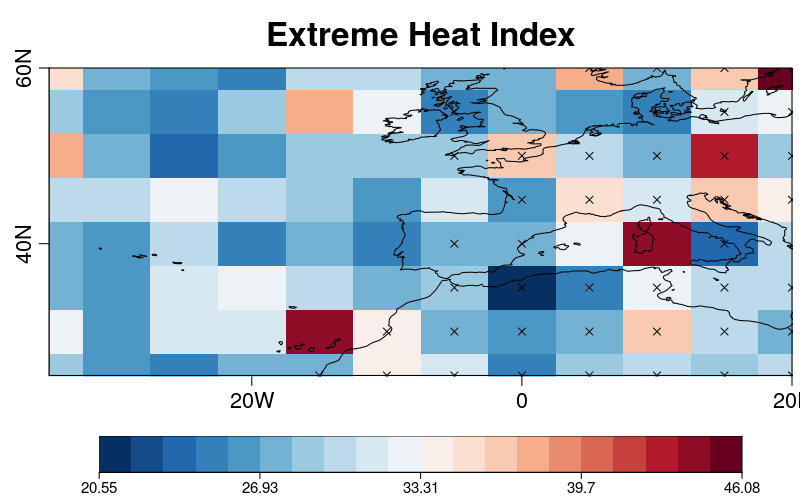The inland average of the Extreme Heat Index can be computed to plot its time evolution using WeigthedMean function. Smoothing() returns the smoothed time series for a 3 year moving window which can be modified using runmeanlen parameter.

temporal <- WeightedMean(HeatExtremeIndex, lon = lon, lat = lat, mask = drop(masc))
temporal_3ysmooth <- Smoothing(temporal, runmeanlen = 3, numdimt = 1)

The next code should be run to plot and save the original average and the 3 year smoothed data.

png("Temporal_Inland_ExtremeHeatIndex.png", width = 8, height = 5, units = 'in',
res = 100, type = "cairo")
plot(2006 : 2100, temporal, type = "l", lty = 5, lwd = 2, bty = 'n',
xlab = "Time (years)", ylab = "Extreme Heat Index",
main = "Inland average Extreme Heat Index")
lines(2006 : 2100, temporal_3ysmooth, col = "darkgreen", lwd = 2)
legend('bottomright', c('Anual', '3 years smooth'), col = c(1, 'darkgreen'),
lty = c(5, 1), lwd = 2, bty = 'n')
dev.off()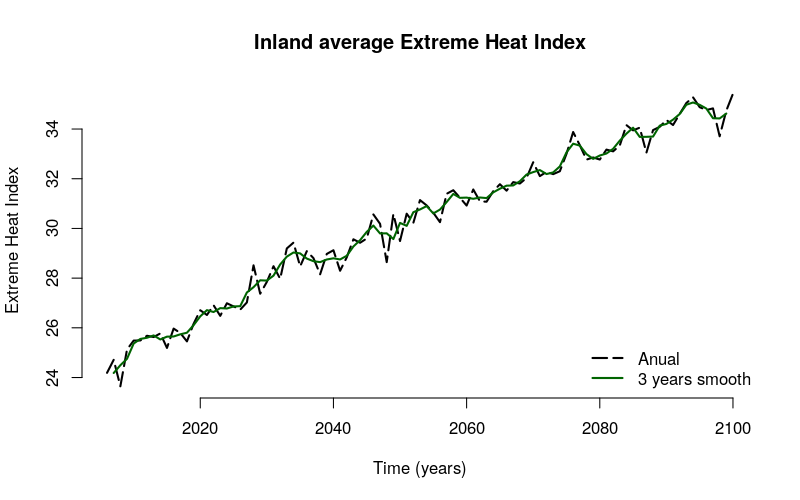### 4- Extreme Drought Index

The Extreme Drought Index (cdd), which measures the maximum length of a dry spell, is defined as the maximum number of consecutive days with the daily precipitation amount lower than 1 mm.

To compute the Extreme Drought Index during the reference period and its standard deviation and mean:

Note: Precipitation data is not detrended. Furthermore, this index doesn’t require to compute a threshold as Climdex function integrates the threshold of precipitation amount lower than 1 mm internally. However, this case requires the calculation of the mean.

base_index <- Climdex(data = ppt_historical, metric = 'cdd',
ncores = detectCores() - 1)
base_mean <-  Apply(list(base_index$result), target_dims = list(c(1)), fun = "mean")$output1
base_sd <- Apply(list(base_index$result), target_dims = list(c(1)), fun = "sd")$output1

The object base_index contains the output of the Climdex function as two list with the next dimensions:

> str(base_index)
List of 2
$result: num [1:30, 1, 1, 1:12, 1:8] 6 11 8 8 8 12 9 10 6 8 ...$ years : num [1:30] 1971 1972 1973 1974 1975 ...

The Extreme Drought Index is computed and standardized:

projection_index <- Climdex(data = ppt_projection, metric = 'cdd',
ncores = detectCores() - 1)
base_mean <- InsertDim(base_mean, 1, dim(projection_index$result)) base_sd <- InsertDim(base_sd, 1, dim(projection_index$result))
DroughtExtremeIndex <- (projection_index$result - base_mean) / base_sd Spatial representation of the Extreme Drought Index: PlotEquiMap(Mean1Dim(DroughtExtremeIndex, which(names(dim(DroughtExtremeIndex)) == "year")), lon = lon, lat = lat, filled.continents = FALSE, toptitle = "Drought Index", brks = seq(-1, 1, 0.01), fileout = "SpatialDroughtIndex.png")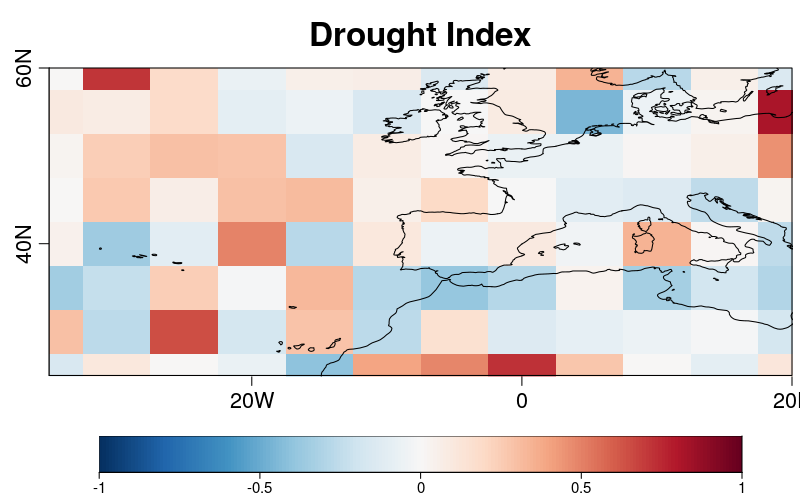Evolution of inland average of the Extreme Drought Index: temporal <- WeightedMean(DroughtExtremeIndex, lon = lon, lat = lat, mask = drop(masc)) temporal_5ysmooth <- Smoothing(temporal, runmeanlen = 5, numdimt = 1) png("Temporal_Inland_ExtremeDroughtIndex.png", width = 8, height = 5, units= 'in', res = 100, type = "cairo") plot(2006: 2100, temporal, type = "l", lty = 5, lwd = 2, bty = 'n', xlab = "Time (years)", ylab = "Extreme Drought Index", main = "Inland average Extreme Drought Index") lines(2006 : 2100, temporal_5ysmooth, col = "darkgreen",lwd = 2) legend('bottomleft', c('Anual', '3 years smooth'), col= c(1, 'darkgreen'), lty = c(5, 1), lwd = 2, bty = 'n') dev.off()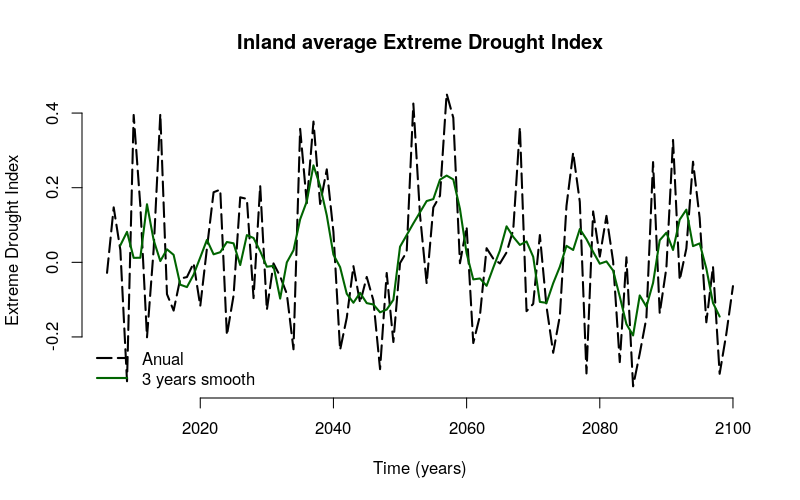### 5- Extreme Flooding Index The Extreme Flooding Index (rx5day) is defined as the maximum precipitation amount in 5 consecutive days. The Extreme Flooding Index during the reference period and its standard deviation and mean can be calculated by executing: base_index <- Climdex(data = ppt_historical, metric = 'rx5day', ncores = detectCores() - 1) base_mean <- Apply(list(base_index$result), target_dims = list(c(1)),
fun = "mean")$output1 base_sd <- Apply(list(base_index$result), target_dims = list(c(1)),
fun = "sd")$output1 The Extreme Flooding Index is computed and standardized: projection_index <- Climdex(data = ppt_projection, metric = 'rx5day', ncores = detectCores() - 1) base_mean <- InsertDim(base_mean, 1, dim(projection_index$result))
base_sd <- InsertDim(base_sd, 1, dim(projection_index$result)) FloodingExtremeIndex <- (projection_index$result - base_mean) / base_sd

Spatial representation of the Extreme Flooding Index:

PlotEquiMap(Mean1Dim(FloodingExtremeIndex,
which(names(dim(FloodingExtremeIndex)) == "year")), lon = lon,
lat = lat, filled.continents = FALSE,
toptitle = "Extreme Flooding Index",
brks = seq(-1, 1, 0.1), fileout = "SpatialFloodingIndex.png")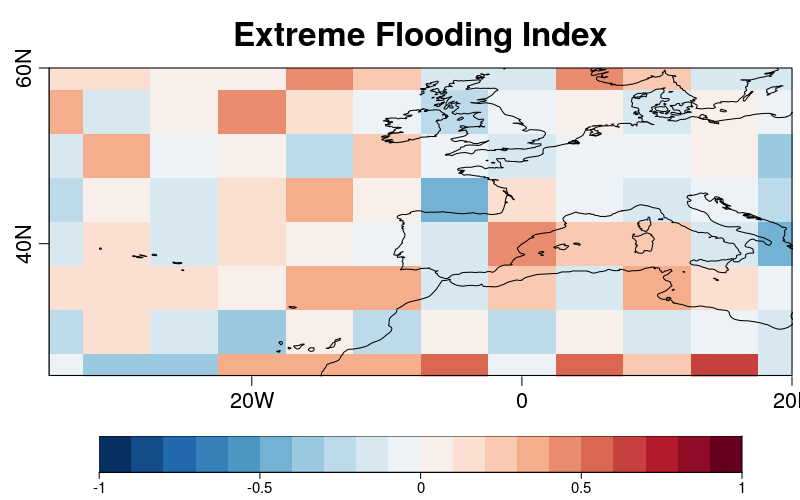• Evolution of inland average of the Extreme Flooding Index:
temporal <- WeightedMean(FloodingExtremeIndex, lon = lon, lat = lat,
temporal_3ysmooth <- Smoothing(temporal, runmeanlen = 3, numdimt = 1)
png("Temporal_Inland_ExtremeFloodingIndex.png", width = 8, height = 5,
units= 'in', res = 100, type = "cairo")
plot(2006 :  2100, temporal, type = "l", lty = 5, lwd = 2, bty = 'n',
xlab = "Time (years)", ylab = "Extreme Flooding Index",
main = "Inland average Extreme Flooding Index")
lines(2006 :  2100, temporal_3ysmooth, col = "darkgreen",lwd = 2)
legend('bottomleft', c('Anual', '3 years smooth'), col= c(1, 'darkgreen'),
lty = c(5, 1), lwd = 2, bty = 'n')
dev.off()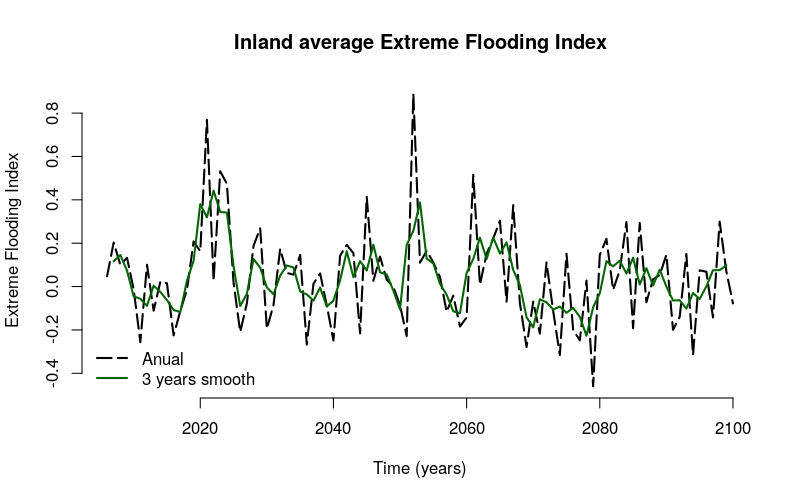### 6- Combining Indices

The individual indices can be combined into a single index with or without weighting for each component. This combined index is roughly analogous to the Actuaries Climate Risk Index. Extreme Indices should be saved in the same list object.

indices <- list()
indices[] <- HeatExtremeIndex
indices[] <- DroughtExtremeIndex
indices[] <- FloodingExtremeIndex

If the weights parameter is defined as NULL, all indices will be equally weighted if the operation parameter is set as mean (by default). To define other weights a vector of length equal to the number of considered indices (5 in this example) and with total sum equal to 1.

aci <- CombineIndices(indices = indices, weights = NULL)

A spatial visualization can be performed by executing:

PlotEquiMap(Mean1Dim(aci, which(names(dim(aci)) == "year")), lon = lon,
lat = lat,  filled.continents = FALSE, toptitle = "Indices Combination",
fileout = "CombinedIndices.png")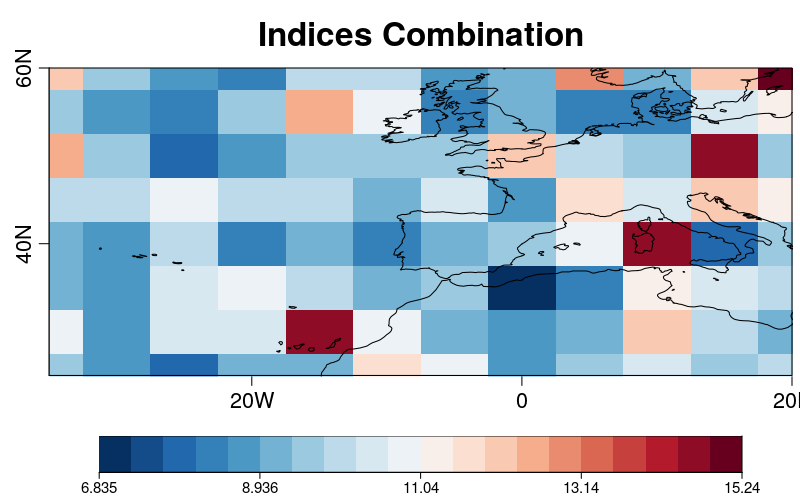Note: This vignette shows the computation of three indices, however, five different indices can be computed with the Climdex function. To consider other combination settings run ?CombinedIndices.